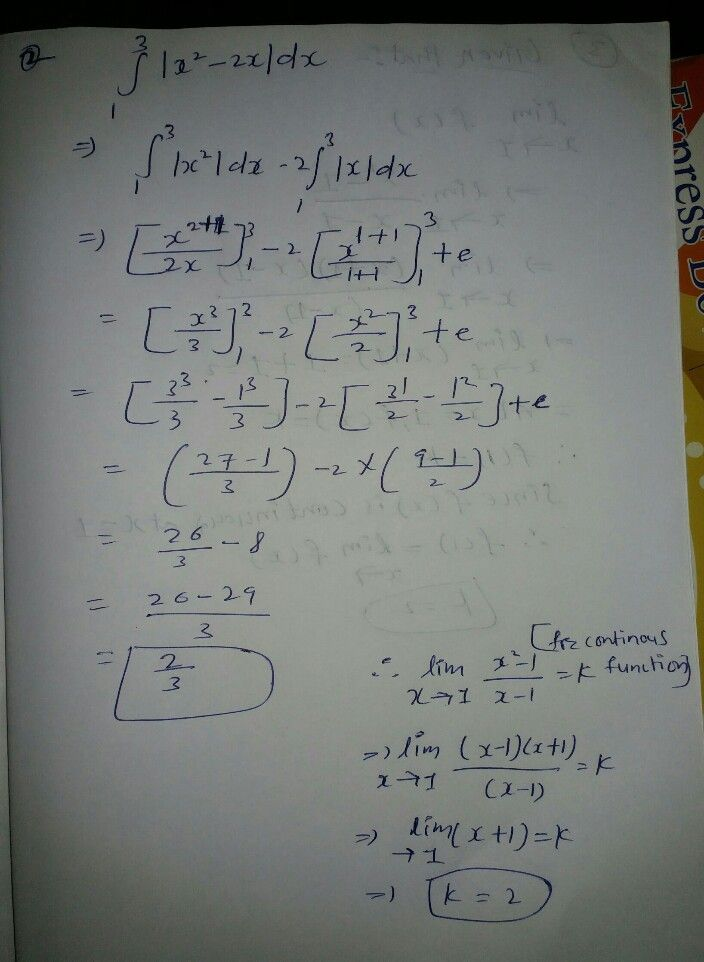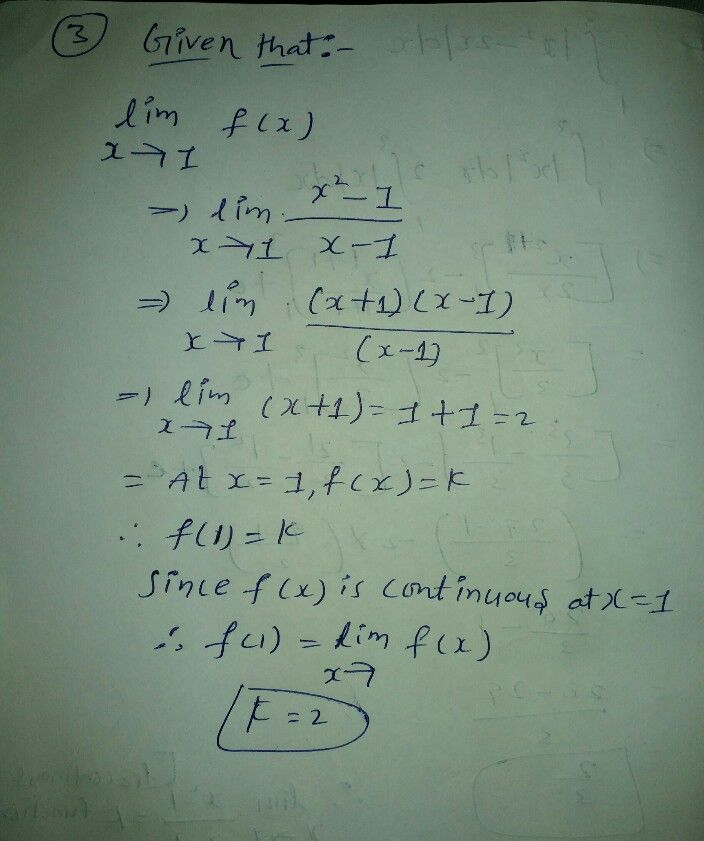Symbol
Problem$sθ=\dfrac {1} {\sqrt{3} }$ then evaluate $1-cos^{2}θ$ $-=-$ $2-sin^{2}θ$ luate $\int ^{3}|x^{2}-2x|dx$ $1$ $f\left(x\right)=\left(\dfrac {x^{2-1}} {x-1}$ $k$ when $x≠$ ie function when $x=$ iven to be continuous at $x=1$
10th-13th grade
Other
Search count: 1,540
SolutionQanda teacher - sweetie